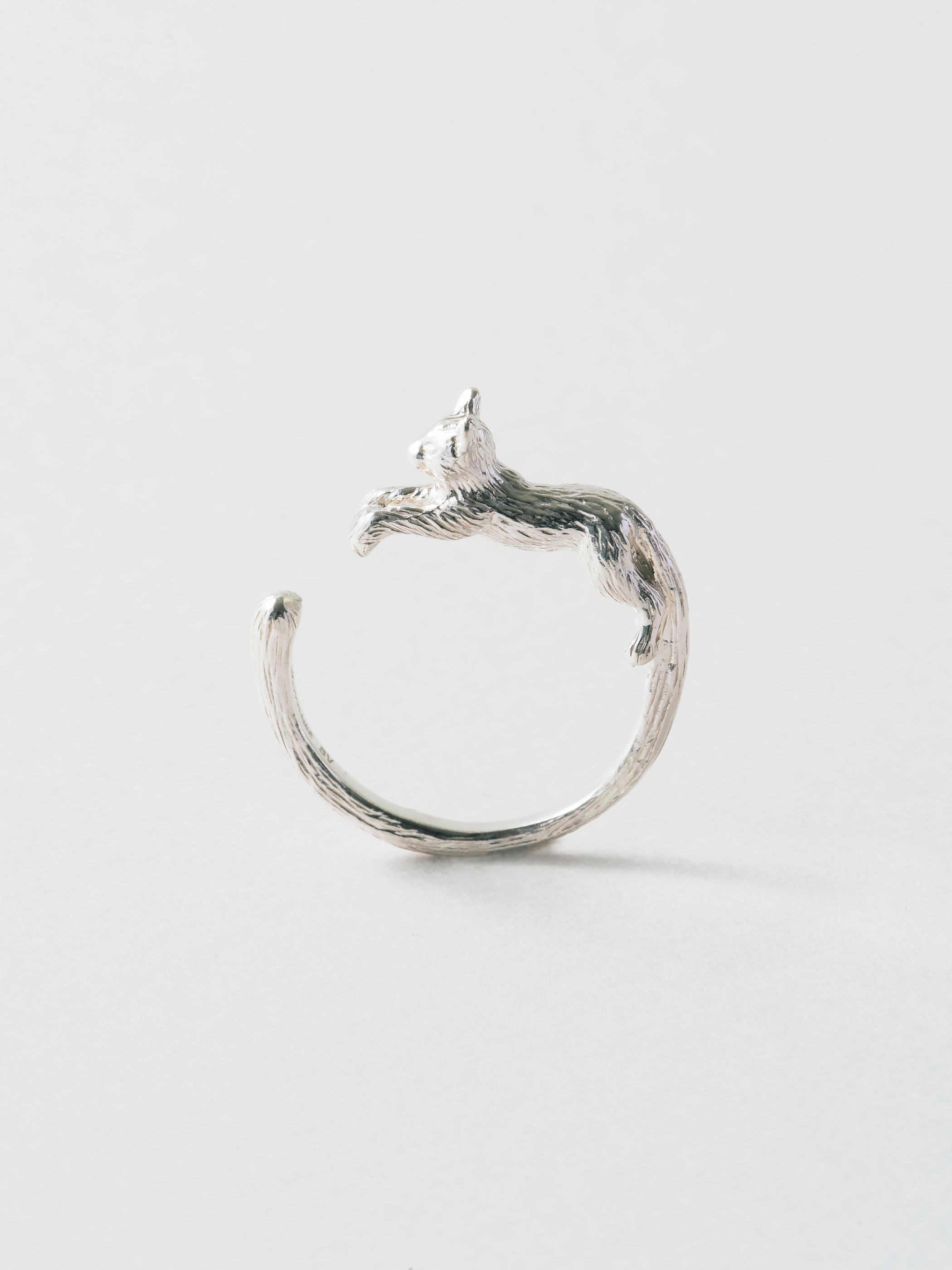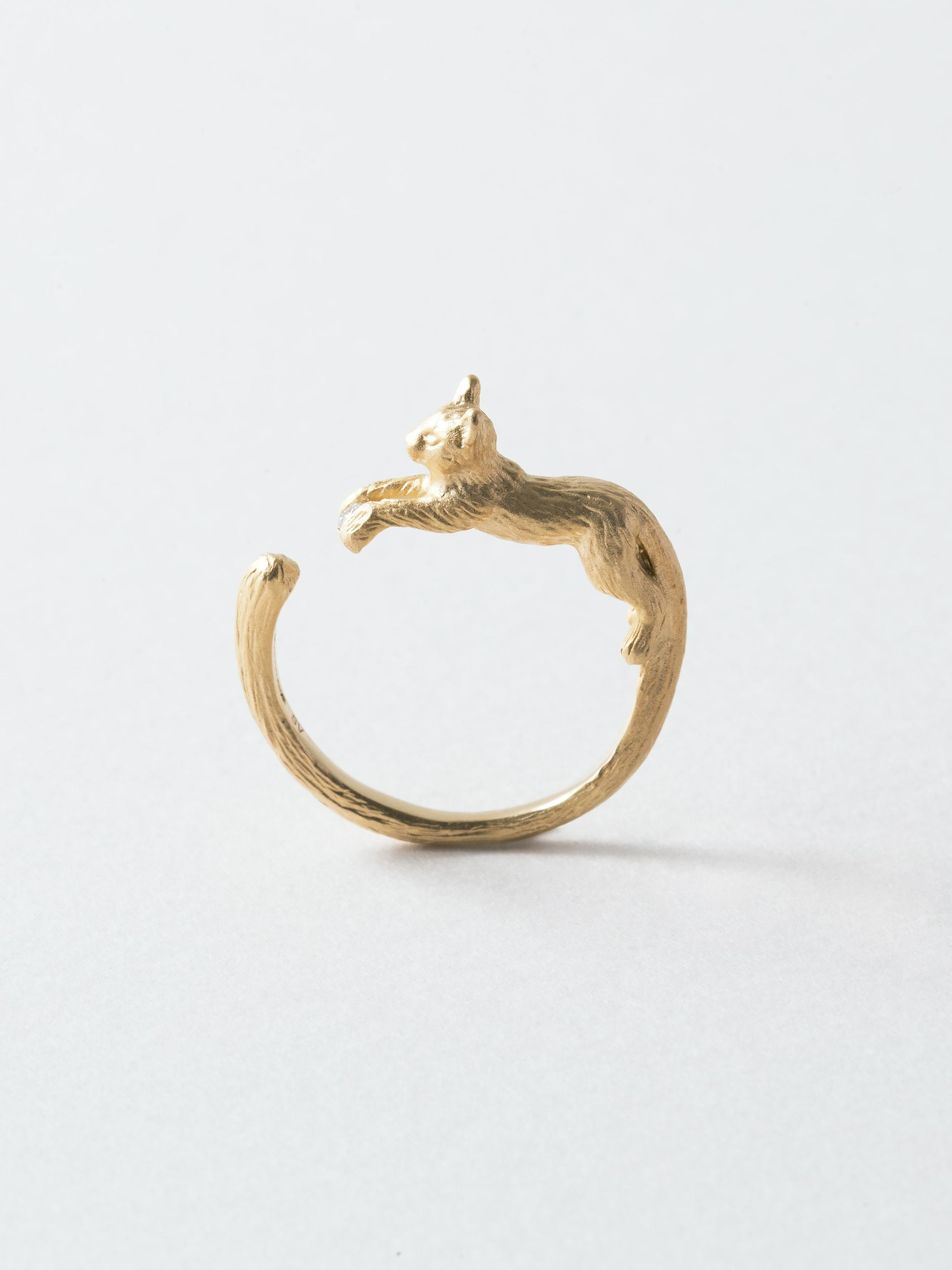Industrial

•••CAT DIAMOND RING

the Ring

DIAMOND:0.02ct

MATERIALS

silver:SILVER 925/DIAMOND(0.02ct)
gold:SILVER 925 (K18Gold Plated)/DIAMOND(0.02ct)

NOTES

RING SIZE GUIDE
JP #7 =US 4-4.5 =UK H-I =EU 47
JP #8 =US 4.5-5 =UK I-J =EU 48
JP #9 =US 5 =UK J =EU 49
JP #10 =US 5.5 =UK K =EU 50
JP #11 =US 6 =UK L =EU 51
JP #12 =US 6.5 =UK L-M =EU 52
JP #13 =US 6.5-7 =UK M-N =EU 53
JP #14 =US 7-7.5 =UK N-O =EU 54
JP #15 =US 7.5-8 =UK O-P =EU 55
JP #16 =US 8 =UK P =EU 56
JP #17 =US 8.5 =UK Q =EU 57

Select the type